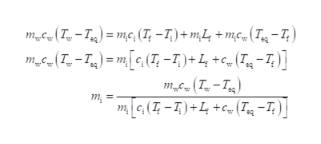# An insulated beaker with negligible mass contains liquid water with a mass of 0.255 kg and a temperature of 63.1 ∘C .How much ice at a temperature of -24.1 ∘C must be dropped into the water so that the final temperature of the system will be 21.0 ∘C ?Take the specific heat of liquid water to be 4190 J/kg⋅K , the specific heat of ice to be 2100 J/kg⋅K , and the heat of fusion for water to be 3.34×105 J/kg .

Question
4 views

An insulated beaker with negligible mass contains liquid water with a mass of 0.255 kg and a temperature of 63.1 ∘C .

How much ice at a temperature of -24.1 ∘C must be dropped into the water so that the final temperature of the system will be 21.0 ∘C ?

Take the specific heat of liquid water to be 4190 J/kg⋅K , the specific heat of ice to be 2100 J/kg⋅K , and the heat of fusion for water to be 3.34×105 J/kg .
check_circle

Step 1

Write an expression equating heat lost by w...help_outlineImage Transcriptionclosem(T-T= me, (T, -7)+ m4 +mco(Tg -7) mc(T-T)= m[G(T, -T)+ L +c_(T«g =7; ] m (T-Tg) m(-T)+L+(T -7;) m fullscreen

### Want to see the full answer?

See Solution

#### Want to see this answer and more?

Solutions are written by subject experts who are available 24/7. Questions are typically answered within 1 hour.*

See Solution
*Response times may vary by subject and question.
Tagged in

### Heat Transfer